Download Presentation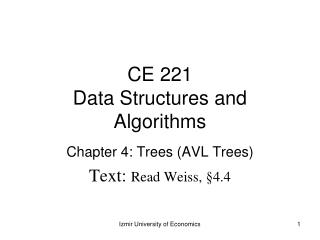CE 221 Data Structures and Algorithms

# CE 221 Data Structures and Algorithms - PowerPoint PPT Presentation

CE 221 Data Structures and Algorithms. Chapter 4: Trees (AVL Trees) T ext : Read Weiss, § 4 . 4. AVL Trees.I am the owner, or an agent authorized to act on behalf of the owner, of the copyrighted work described.
Download Presentation## CE 221 Data Structures and Algorithms

An Image/Link below is provided (as is) to download presentation

Download Policy: Content on the Website is provided to you AS IS for your information and personal use and may not be sold / licensed / shared on other websites without getting consent from its author.While downloading, if for some reason you are not able to download a presentation, the publisher may have deleted the file from their server.

- - - - - - - - - - - - - - - - - - - - - - - - - - E N D - - - - - - - - - - - - - - - - - - - - - - - - - -
Presentation Transcript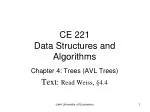### CE 221Data Structures and Algorithms

Chapter 4: Trees (AVL Trees)

Text: Read Weiss, §4.4

Izmir University of Economics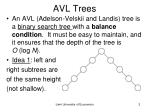AVL Trees
• An AVL (Adelson-Velskii and Landis) tree is a binary search tree with a balance condition. It must be easy to maintain, and it ensures that the depth of the tree is O (log N).
• Idea 1: left and

right subtrees are

of the same height

(not shallow).

Izmir University of Economics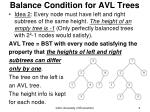Balance Condition for AVL Trees
• Idea 2: Every node must have left and right subtrees of the same height. The height of an empty tree is -1 (Only perfectly balanced trees with 2k-1 nodes would satisfy).

AVL Tree = BST with every node satisfying the

property that the heights of left and right

subtrees can differ

only by one.

The tree on the left is

an AVL tree.

The height info is kept

for each node.

Izmir University of Economics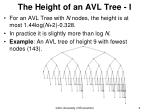The Height of an AVL Tree - I
• For an AVL Tree with N nodes, the height is at most 1.44log(N+2)-0.328.
• In practice it is slightly more than log N.
• Example: An AVL tree of height 9 with fewest nodes (143).

Izmir University of Economics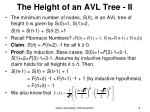The Height of an AVL Tree - II
• The minimum number of nodes, S(h), in an AVL tree of height h is given by S(0)=1, S(1)=2,

S(h) = S(h-1) + S(h-2) +1

• Recall Fibonacci Numbers? (F(0) = F(1) = 1, F(n)=F(n-1) + F(n-2))
• Claim: S(h) = F(h+2) -1 for all h ≥ 0.
• Proof: By induction. Base cases; S(0)=1=F(2)-1=2-1, S(1)=2=F(3)-1=3-1. Assume by inductive hypothesis that claim holds for all heights k ≤ h. Then,
• S(h+1) = S(h) + S(h-1) + 1

= F(h+2) -1 +F(h+1) -1 + 1 (by inductive hypothesis)

= F(h+3) – 1

• We also know that

Izmir University of Economics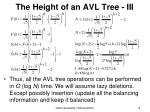The Height of an AVL Tree - III
• Thus, all the AVL tree operations can be performed in O (log N) time. We will assume lazy deletions. Except possibly insertion (update all the balancing information and keep it balanced)

Izmir University of Economics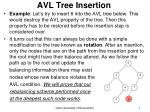AVL Tree Insertion
• Example: Let’s try to insert 6 into the AVL tree below. This would destroy the AVL property of the tree. Then this property has to be restored before the insertion step is considered over.
• It turns out that this can always be done with a simple modification to the tree known as rotation. After an insertion, only the nodes that are on the path from the insertion point to the root might have their balance altered. As we follow the path up to the root and update the

balancing information there may exist

nodes whose new balance violates the

AVL condition. We will prove that our

rebalancing scheme performed once

at the deepest such node works.

6

Izmir University of Economics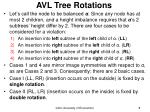AVL Tree Rotations

• Let’s call the node to be balanced α. Since any node has at most 2 children, and a height imbalance requires that α‘s 2 subtrees’ height differ by 2. There are four cases to be considered for a violation:
• An insertion into left subtree of the left child of α. (LL)
• An insertion into right subtree of the left child of α. (LR)
• An insertion into left subtree of the right child of α. (RL)
• An insertion into right subtree of the right child of α. (RR)
• Cases 1 and 4 are mirror image symmetries with respect to α, as are Cases 2 and 3. Consequently; there are 2 basic cases.
• Case I (LL, RR) (insertion occurs on the outside) is fixed by a single rotation.
• Case II (RL, LR) (insertion occurs on the inside) is fixed by double rotation.

Izmir University of Economics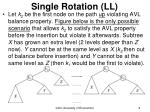Single Rotation (LL)
• Let k2 be the first node on the path up violating AVL balance property. Figure below is the only possible scenario that allows k2 to satisfy the AVL property before the insertion but violate it afterwards. Subtree X has grown an extra level (2 levels deeper than Z now). Y cannot be at the same level as X (k2 then out of balance before insertion) and Y cannot be at the same level as Z (then k1 would be the first to violate).

Izmir University of Economics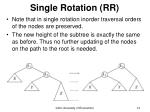Single Rotation (RR)

• Note that in single rotation inorder traversal orders of the nodes are preserved.
• The new height of the subtree is exactly the same as before. Thus no further updating of the nodes on the path to the root is needed.

Izmir University of EconomicsSingle Rotation-Example I

• AVL property destroyed by insertion of 6, then fixed by a single rotation.
• BST node structure needs an additional field for height.

Izmir University of EconomicsSingle Rotation-Example II

• Start with an initially empty tree and insert items 1 through 7 sequentially. Dashed line joins the two nodes that are the subject of the rotation.

Izmir University of EconomicsSingle Rotation-Example III

Insert 6. Balance problem at the root. So a single rotation is performed.

Finally, Insert 7 causing another rotation.

Izmir University of EconomicsDouble Rotation (LR, RL) - I
• The algorithm that works for cases 1 and 4 (LL, RR) does not work for cases 2 and 3 (LR, RL). The problem is that subtree Y is too deep, and a single rotation does not make it any less deep.
• The fact that subtree Y has had an item inserted into it guarantees that it is nonempty. Assume it has a root and two subtrees.

Izmir University of EconomicsDouble Rotation (LR) - II

Below are 4 subtrees connected by 3 nodes. Note that exactly one of tree B or C is 2 levels deeper than D (unless all empty). To rebalance, k3 cannot be root and a rotation between k1 and k3 was shown not to work. So the only alternative is to place k2 as the new root. This forces k1 to be k2’s left child and k3 to be its right child. It also completely determines the locations of all 4 subtrees. AVL balance property is now satisfied. Old height of the tree is restored; so, all the balancing and and height updating is complete.

Izmir University of EconomicsDouble Rotation (RL) - III

In both cases (LR and RL), the effect is the same as rotating between α’s child and grandchild and then between αand its new child. Every double rotation can be modelled in terms of 2 single rotations. Inorder traversal orders are always preserved between k1, k2, and k3.

Double RL = Single LL (α->right)+ Single RR (α)

Double LR = Single RR (α->left)+ Single LL (α )

Izmir University of EconomicsDouble Rotation Example - I
• Continuing our example, suppose keys 8 through 15are inserted in reverse order. Inserting 15 is easy but inserting 14 causes a height imbalance at node 7. The double rotation is anRLtype and involves 7, 15, and 14.

Izmir University of EconomicsDouble Rotation Example - II
• insert 13: double rotation is RL that will involve 6, 14, and 7 and will restore the tree.

Izmir University of EconomicsDouble Rotation Example - III

• If 12 is now inserted, there is an imbalance at the root. Since 12 is not between 4 and 7, we know that the single rotation RR will work.

Izmir University of EconomicsDouble Rotation Example - IV

• Insert 11: single rotation LL; insert 10: single rotation LL; insert 9: single rotation LL; insert 8: without a rotation.

Izmir University of EconomicsDouble Rotation Example - V

• Insert 8½:double rotation LR. Nodes 8, 8½, 9 are involved.

Izmir University of EconomicsImplementation Issues - I
• To insert a new node with key X into an AVL tree T, we recursively insert X into the appropriate subtree of T (let us call this TLR). If the height of TLR does not change, then we are done. Otherwise, if a height imbalance appears in T, we do the appropriate single or double rotation depending on X and the keys in T and TLR, update the heights (making the connection from the rest of the tree above), and are done.

Izmir University of EconomicsImplementation Issues - II
• Another efficiency issue concerns storage of the height information. Since all that is really required is the difference in height, which is guaranteed to be small, we could get by with two bits (to represent +1, 0, -1) if we really try. Doing so will avoid repetitive calculation of balance factors but results in some loss of clarity. The resulting code is somewhat more complicated than if the height were stored at each node.

Izmir University of EconomicsImplementation Issues - III
• First, the declarations. Also, a quick function to return the height of a node dealing with the annoying case of a NULL pointer.

typedef int ElementType;

struct AvlNode;

typedef struct AvlNode *Position;

typedef struct AvlNode *AvlTree;

struct AvlNode {

ElementType Element;

AvlTree Left;

AvlTree Right;

int Height;

};

static int Height( Position P ) {

if( P == NULL )

return -1;

else

return P->Height;

}

Izmir University of EconomicsImplementation - LL

/* This function can be called only if K2 has */

/* a left child. Perform a rotate between */

/* a node (K2)and its left child. Update heights, */

/* then return new root */

static Position SingleRotateWithLeft( Position K2 ){

Position K1;

K1 = K2->Left;

K2->Left = K1->Right;

K1->Right = K2;

K2->Height=Max(Height(K2->Left), Height(K2->Right))+1;

K1->Height=Max(Height(K1->Left), K2->Height)+1;

return K1; /* New root */

}

Izmir University of EconomicsImplementation - RR

/* This function can be called only if K1 has */

/* a right child. Perform a rotate between */

/* a node (K1) and its right child. Update heights, */

/* then return new root */

static Position SingleRotateWithRight( Position K1 ) {

Position K2;

K2 = K1->Right;

K1->Right = K2->Left;

K2->Left = K1;

K1->Height=Max(Height(K1->Left), Height(K1->Right))+1;

K2->Height=Max(Height(K2->Right), K1->Height)+1;

return K2; /* New root */

}

Izmir University of EconomicsImplementation - LR

/* Double LR = Single RR (α->left)+ Single LL (α ) */

/* This function can be called only if K3 has a left */

/* child and K3's left child has a right child */

/* Do the left-right double rotation */

/* Update heights, then return new root */

static Position DoubleRotateWithLeft( Position K3 ){

/* Rotate between K1 and K2 */

K3->Left = SingleRotateWithRight( K3->Left );

/* Rotate between K3 and K2 */

return SingleRotateWithLeft( K3 );

}

Izmir University of EconomicsImplementation - RL

/* Double RL = Single LL (α->right)+ Single RR (α) */

/* This function can be called only if K1 has a right */

/* child and K1's right child has a left child */

/* Do the right-left double rotation */

/* Update heights, then return new root */

static Position DoubleRotateWithRight( Position K1 ){

/* Rotate between K3 and K2 */

K1->Right = SingleRotateWithLeft( K1->Right );

/* Rotate between K1 and K2 */

return SingleRotateWithRight( K1 );

}

Izmir University of EconomicsAvlTree Insert( ElementType X, AvlTree T ){

if( T == NULL ){

/* Create and return a one-node tree */

T = malloc( sizeof( structAvlNode ) );

if( T == NULL )

FatalError( "Out of space!!!" );

else {

T->Element = X; T->Height = 0;

T->Left = T->Right = NULL;

}

}

else if( X < T->Element ){

T->Left = Insert( X, T->Left );

if( Height( T->Left ) - Height( T->Right ) == 2 )

if( X < T->Left->Element )

T = SingleRotateWithLeft( T ); /* LL */

else

T = DoubleRotateWithLeft( T ); /* LR */

} /* continued on the next slide */ /* ... */

Implementation Insertion - I

Izmir University of EconomicsImplementation – Insertion II

else if( X > T->Element ){

T->Right = Insert( X, T->Right );

if( Height( T->Right ) - Height( T->Left ) == 2 )

if( X > T->Right->Element )

T = SingleRotateWithRight( T ); /* RR */

else

T = DoubleRotateWithRight( T ); /* RL */

}

/* Else X is in the tree already; we'll do nothing */

T->Height=Max(Height(T->Left),Height(T->Right))+1;

return T;

}

Implementation Insertion - II

Izmir University of EconomicsAVL Tree Deletion* - I

AvlTree Delete( ElementType X, AvlTree T ){

if( T == NULL )

else if( X < T->Element ){

T->Left = Delete( X, T->Left );

if( Height( T->Left ) - Height( T->Right ) == -2 )

if( Height(T->Right->Right) > Height(T->Right->Left) )

T = SingleRotateWithRight( T );/* RR */

else

T = DoubleRotateWithRight( T ); /* RL */

}

else if( X > T->Element ){

T->Right = Delete( X, T->Right );

if( Height( T->Right ) - Height( T->Left ) == -2 )

if( Height(T->Left->Left) > Height(T->Left->Right) )

T = SingleRotateWithLeft( T );/* LL */

else

T = DoubleRotateWithLeft( T ); /* LR */

} /* Continued on the next slide */ /* ... */

Izmir University of EconomicsAVL Tree Deletion* - II

else if( T->Left && T->Right ){/* Found with two children */

/* Replace with smallest in right subtree */

TmpCell = FindMin( T->Right );

T->Element = TmpCell->Element;

T->Right = Delete( T->Element, T->Right );

if( Height( T->Right ) - Height( T->Left ) == -2 )

if( Height(T->Left->Left) > Height(T->Left->Right) )/*LL*/

T = SingleRotateWithLeft( T );

else

T = DoubleRotateWithLeft( T ); /*LR*/

}

else {/* Found with one or zero child */

TmpCell = T;

T = T->Left ? T->Left : T->Right; /* Also handles 0 child */

free( TmpCell );

}

if( T!= NULL )

T->Height=Max(Height(T->Left), Height(T->Right))+1;

return T;

}

Izmir University of EconomicsHomework Assignments
• 4.18, 4.19, 4.25
• You are requested to study and solve the exercises. Note that these are for you to practice only. You are not to deliver the results to me.

Izmir University of Economics